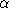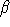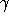CGAL::make_root_of_2

Definition

The function make_root_of_2 constructs an algebraic number of degree 2 over a ring number type.

#include <CGAL/Root_of_traits.h>

template <typename RT>
Root_of_traits<RT>::RootOf_2 make_root_of_2 ( RT a, RT b, RT c, bool s)
Returns the real root of the polynomial aX2+bX+c which is the smallest if s is true, or the largest if s is false.
 Precondition: RT is a RingNumberType.
 Precondition: The polynomial has at least one real root.

template <typename RT>
Root_of_traits<RT>::RootOf_2 make_root_of_2 ( RT alpha, RT beta, RT gamma)
Constructs the number+sqrt().
 Precondition: RT is a RingNumberType.
 Precondition:0